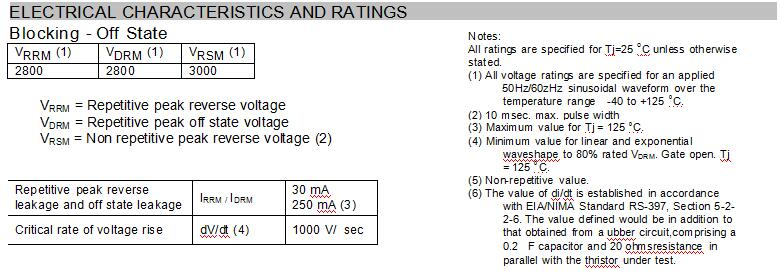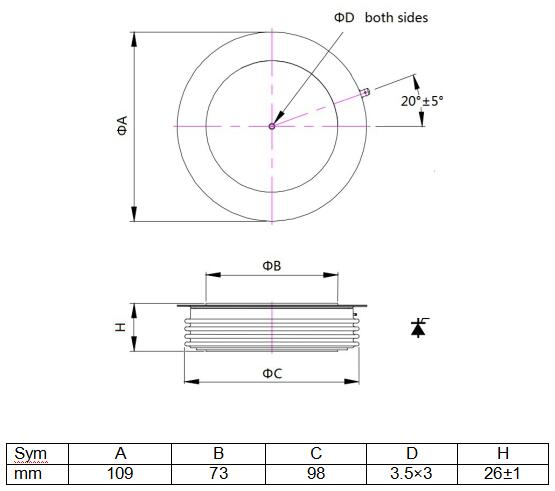# 2800V High power thyristor for power converter

• YZPST-5STP24L2800

High Power Thyristor Phase Control

YZPST-5STP24L2800

HIGH POWER THYRISTOR FOR PHASE CONTROL FEATURES AND APPLICATIONS

Features:

. All Diffused Structure

. Interdigitated Amplifying Gate Configuration

. Guaranteed Maximum Turn-Off Time

. High dV/dt  Capability

. Pressure Assembled Device""

Conducting - on state

 Parameter Symbol Min. Max. Typ. Units Conditions Average value of on-state current IT(AV)M 2400 A Sinewave,180oconduction,T =85oCc RMS value of on-state current ITRMSM 4120 A Nominal value Peak one cPSTCle surge(non repetitive) current ITSM 46 43 kA kA 8.3 msec (60Hz), sinusoidal wave-shape, 180o  conduction, T = 125joC10.0 msec (50Hz), sinusoidal wave-shape, 180o  conduction, T = 125joC I square t I2t 8.7x106 A2s 8.3 msec Latching current IL 200 mA VD  = 24 V; RL= 12 ohms Holding current IH 75 mA VD = 24 V; I = 2.5 A Peak on-state voltage VTM 1.35 V I  = 3000 A; T = 125 oCTM  j Threshold vlotage VT0 0.85 V Slope resistance rT 0.16 mΩ Critical rate of rise of on-state current (5, 6) di/dt 300 A/  s Switching from VDRM 1000 V, non-repetitive Critical rate of rise of on-state current (6) di/dt 150 A/  s Switching from VDRM  1000 V

Gating

 Parameter Symbol Min. Max. Typ. Units Conditions Peak gate power dissipation PGM - W Average gate power dissipation PG(AV) - W Peak gate current IGM - A Gate current required to trigger all units IGT 400 mA V   = 10 V;I =3A;T = +25 oCD  T  j Gate voltage required to trigger all units VGT 2.6 V V   = 10 V;I =3A;T = +25 oCD  T  j Peak negative voltage VRGM - V

Dynamic

 Parameter Symbol Min. Max. Typ. Units Conditions Delay time tgd 3 - s VD=67% VDRM, IT=2000A, di/dt=60A/us, IFG=2A, tr=0.5us, Tj=25C Turn-on time tgt - - Turn-off time (with VR  = -5 V) tq - - 400 s ITM=1000A, tp=1000us, di/dt=60A/us, Vr=50V, Vdr=80%VDRM, dVdr/dt=20V/us Reverse recovery current Irm - A ITM=4000A, tp=2000us, di/dt=60A/us

THERMAL AND MECHANICAL CHARACTERISTICS AND RATINGS

 Parameter Symbol Min. Max. Typ. Units Conditions Operating temperature Tj -40 +125 oC Storage temperature Tstg -40 +140 oC Thermal resistance - junction tocase R  (j-c) 1020 K/kW Double sided cooled *Single sided cooled * Thermal resistamce - case to sink R  (c-s) 24 K/kW Double sided cooled * Single sided cooled * Thermal resistance - junction to case R  (j-s) -- K/kW Double sided cooled * Single sided cooled * Mounting force F 45 60 50 kN Weight W 0.9 Kg about

* Mounting surfaces smooth, flat and greased

Note : for case outline and dimensions, see case outline drawing in last page of this Technical Data

Outline""